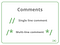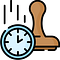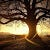# Integer To English Words (Leetcode 273)

This is a comprehensive walkthrough for the solution to the problem Integer to English Words problem mentioned on Leetcode.

# Problem Description

The question is basically to come up with a way to convert any non negative integer to its English words representation.

eg. 123 is written as “One Hundered Twenty Three”

# Approach

The approach we are gonna follow is to divide the number in a set of three like we normally do while writing numbers starting from left to right in sequence one, tens, hundred, thousand, ten thousand, hundred thousand, million, ten million, hundred million, billion and so on.

The flow starts from…

# Course Schedule

This is the solution for the Leetcode problem Course Schedule. With a little modification we can also solve Couse Schedule II , where we need to order the courses in the manner of their dependencies.

# Problem Statement

There are a total of `numCourses` courses you have to take, labeled from `0` to `numCourses - 1`. You are given an array `prerequisites` where `prerequisites[i] = [ai, bi]` indicates that you must take course `bi` first if you want to take course `ai`.

• For example, the pair `[0, 1]`, indicates that to take course `0` you have to first take course `1`.

Return `true`

# House Robber II

This is a follow up question to the House Robber problem on Leetcode. Please go through the explanation for the original problem here (https://nishantt.medium.com/house-robber-afe8131a5abb) before proceeding ahead.

The only change here is that the houses are now placed in a circular fashion i.e. the last house is the neighbor to the first house and vice versa. Hence the robber can now only rob either the last house or the first house in one go as robbing one would alert the other.

# Recursion

Our approach here will be a modification of the original House Robber problem. So we are gonna call our…

# House Robber

This is the solution for House Robber Problem on Leetcode.

# Problem Description

You are a professional robber planning to rob houses along a street. Each house has a certain amount of money stashed, the only constraint stopping you from robbing each of them is that adjacent houses have security systems connected and it will automatically contact the police if two adjacent houses were broken into on the same night.

Given an integer array `nums` representing the amount of money of each house, return the maximum amount of money you can rob tonight without alerting the police.

# Solution

## Recursive Approach :

Our approach here will be to…

# Unique Binary Search Trees

This is the solution to the unique binary search tree problem on Leetcode.

Here the logic is that for each index i in n we are gonna calculate the unique trees for i-1 and n-i nodes and multiply them. Eventually adding the number of trees for all indices. The problem boils down to that, for n = 0 and n = 1 the total number of unique binary trees are 1. The recursive solution makes use of this logic as it is.

# Recursive Solution Runtime — 2876 ms

Below is the recursive tree for n = 3 which comes out to be 5.Image Credits GeeksforGeeks

# Remove Comments

This is the explanation for the Leetcode question named “Remove Comments”(https://leetcode.com/problems/remove-comments/)

The problem may seems daunting at first and to be honest even I had been avoiding it it for some months now. But decided to give it some time today and sharing my experience. Once you spend enough time on it the logic comes to you as it mostly brute force and does not require prior knowledge of any algorithm or special data structure.

# Logic

The problem boils down to removing comments. There are two kinds of comment strategy in C#. …

# Decode Ways (Leetcode 91)

This is the solution for Leetcode problem number 91 Decode ways(https://leetcode.com/problems/decode-ways/)

I am gonna explain this in parts. Firstly we will start with a recursion solution as it is the most intuitive way of thinking while attempting a DP question. Then we are gonna come with the Dynamic Programming solution using Memoization.

# Recursive Solution

The pattern we are gonna apply here can be further used to calculate the total number of possibilities or choices for any kind of problem. Similar problems are Fibonacci, Staircase etc.

The choices we have is either we are gonna pick the next character and decode it or…

# Robot Bounded In Circle

This is a discussion on Leetcode problem 1041. (https://leetcode.com/problems/robot-bounded-in-circle/)

This code might be a bit lengthy than other solutions found on the internet but much more readable in my view.
So the trick as rightly given in the problem hint is that the robot is moving in a circle if:
1) Either the original direction has been changed i.e. the robot is no longer facing North.
2) Or After all the instructions have been executed robot has returned to it starting position which is (0,0).

Created the Points class to keep track of the location change for the robot, moving…Timestamp based Key-Value Store

# Time Based Key-Value Store

This article talks about the Leetcode problem number 981. Time Based Key-Value Store.(https://leetcode.com/problems/time-based-key-value-store/)

The basic idea here is to store the key value pairs along with the time stamp and retrieve them according to the timestamp.

Storage Part

To store such a structure we create a Cache class with members as timestamp and value.
This Cache object is mapped to the given key using the Dictionary data structure as Dictionary<string, List<Cache>>.
A single key can have multiple values at different timestamps.
We check if there is already an entry against the key, if not we create an entry and initialize our List<Cache> for…